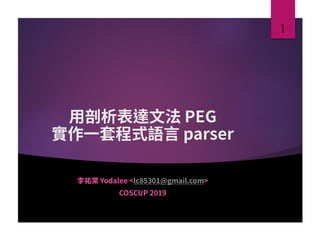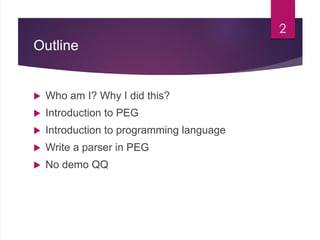Successfully reported this slideshow.

# Use PEG to Write a Programming Language Parser×

# Use PEG to Write a Programming Language Parser

PEG is a replacement to CFG. It is more powerful and can be more precise. In this slide I give a short introduction to PEG, the concept behind a programming language. Finally I write a parser for our programming language simple.

PEG is a replacement to CFG. It is more powerful and can be more precise. In this slide I give a short introduction to PEG, the concept behind a programming language. Finally I write a parser for our programming language simple.

### Use PEG to Write a Programming Language Parser

1. 1. 1
2. 2. Outline  Who am I? Why I did this?  Introduction to PEG  Introduction to programming language  Write a parser in PEG  No demo QQ 2
3. 3. About Me  葉闆, Yodalee <lc85301@gmail.com>  Study EE in college, Microwave in graduate school, now rookie engineer in Synopsys. 3 Github: yodalee Blogger: http://yodalee.blogspot.tw
4. 4. Why Did I Do This  “Understanding Computation: From Simple Machines to Impossible Programs”  In the book, it implements a programming language parser, regular expression parser with Ruby Treetop, which is a PEG parser.  I re-write all the code in Rust, so I did a little research on PEG. https://github.com/yodalee/computationbook -rust 4
5. 5. Introduction to PEG 5
6. 6. Parsing Expression Grammar, PEG  Bryan Ford, <Parsing Expression Grammars: A Recognition- Based Syntactic Foundation>, 2004  A replacement to Chomsky language, by removing the ambiguity in grammar.  The ambiguity is useful in modeling natural language, but not in precise and unambiguous programming language. 6 Language <- Subject Verb Noun Subject <- He | Lisa … Verb <- is | has | sees… Noun <- student | a toy …
7. 7. PEG Basic Rule  PEG in definition are very similar to CFG, composed of rules.  Rule will either:  Match success: consume input.  Match fail: not consume input.  As predicate: only return success or fail, not consume input. 7
8. 8. PEG Basic Rule  Replace choice ‘|’ with prioritized choice ‘/’.  Consider following:  CFG: A = “a” | “ab” PEG: A = “a” / “ab”  PEG: A = a* a 8 Operator “” String Literal [] Character Set . Any Character (e1 e2 ..) Grouping e? e+ e* Optional Repetition &e And predicate !e Not predicate e1 e2 Sequence e1 / e2 Prioritized Choice
9. 9. Some Example  NUMBER <- [1-9] [0-9]*  COMMENT <- “//” (!”n” .)* n  EXPRESSION <- TERM ([+-] TERM)* TERM <- FACTOR ([*/] FACTOR)*  STAT_IF <- “if” COND “then” STATEMENT “else” STATEMENT / “if” COND “then” STATEMENT 9
10. 10. PEG is not CFG  PEG is equivalent to Top Down Programming Language (TDPL)  Language anbncn is not context-free, however PEG can parse it with And-predicate.  In CFG, A <- aAa | a match: odd number “a” In PEG, A <- aAa / a match: 2n-1 “a”  It is an open problem that any CFG can be parsed by PEG 10 A <- aAb / ε B <- bBc / ε S <- &(A !b) a* B
11. 11. Using PEG  There are many library that supports PEG:  Rust: rust-peg, pest, nom-peg …  C++: PEGTL, Boost …  Ruby: kpeg, raabro, Treetop …  Python: pyPEG, parsimonious …  Haskell: Peggy …  …  So why Rust? 11
12. 12. Introduction to Programming Language 12
13. 13. Simple Language  3 types of statements: assign, if else, while.  Support integer arithmetic.  Support pair, list, function with one argument. Simple, but actually we can do some complex things, like recursion, map. 13 factorfun = function factor(x) { if (x > 1) { x * factor ( x-1 ) } else { 1 } } result = factorfun(10); // 3628800 function last(l) { if (isnothing(snd(l))) { fst(l) } else { last(snd(l)) } }
14. 14. Abstract Syntax Tree  Use Rust enum to store a payload inside.  “Programming” like this: 14 pub enum Node { Number(i64), Boolean(bool), Add(Box<Node>, Box<Node>), Subtract(Box<Node>, Box<Node>), LT(Box<Node>, Box<Node>) … } let n = Node::add(Node::number(3), Node::number(4)) Add 3 4 LT 8
15. 15. Abstract Syntax Tree  All the statement are Node: 15 pub enum Node { Variable ( String ), Assign ( String, Box<Node>), If ( Box<Node>, Box<Node>, Box<Node> ), While ( Box<Node>, Box<Node> ), … }
16. 16. Pair, List and Nothing  Node::pair(Node::number(3), Node::number(4))  List [3,4,5] = pair(3, pair(4, pair(5, nothing)))  Nothing special 16 Pair 3 4 Pair 3 Pair 4 Pair Nothing5
17. 17. Environment and Machine  Environment stores a Hashmap<String, Box<Node>>, with <add> and <get> interface.  A machine accepts an AST and an environment to evaluate AST inside the machine. 17 pub struct Environment { pub vars: HashMap<String, Box<Node>> } pub struct Machine { pub environment: Environment, expression: Box<Node> }
18. 18. Evaluate the AST  Add evaluate function to all AST node using trait.  The result will be a new Node. 18 fn evaluate(&self, env: &mut Environment) -> Box<Node>; match *self { Node::Add(ref l, ref r) => { Node::number(l.evaluate(env).value() + r.evaluate(env).value()) } … }
19. 19. Evaluate the AST  How to evaluate While Node ( condition, body )?  Evaluate condition => evaluate body and self if true. 19 x = 3; while (x < 9) { x = x * 2; } Evaluate x = 3 Evaluate while (x < 9) x = x * 2 Evaluate x = x * 2 Evaluate while (x < 9) x = x * 2 Evaluate x = x * 2 Evaluate while (x < 9) x = x * 2
20. 20. Function  Function is also a type of Node. Upon evaluation, function is wrapped into Closure with environment at that time.  Call is evaluated the function with closure’s environment. 20 Node::Func(String, String, Box<Node>) Node::Closure(Environment, Box<Node>) fn evaluate(&self, env: &mut Environment) -> Box<Node> { Node::Fun(ref name, ref arg, ref body) => { Node::closure(env.clone(), Box::new(self.clone())) } }
21. 21. Call a Function fn evaluate(&self, env: &mut Environment) -> Box<Node> { Node::Call(ref closure, ref arg) => { match *closure { Node::Closure(ref env, ref fun) => { if let Node::Fun(funname, argname, body) = *fun.clone() { let mut newenv = env.clone(); newenv.add(&funname, closure.evaluate(env)); newenv.add(&argname, arg.evaluate(env)); body.evaluate(&mut newenv); } } } } } 21
22. 22. Free Variable  Evaluate the free variables in a function to prevent copy whole environment  Node::Variable  Node::Assign  Node::Function 22 function addx(x) { function addy(y) { x + y }} -> no free variables function addy(x) { x + y } -> free variable y
23. 23. Call a Function if let Node::Fun(funname, argname, body) = *fun.clone() { let mut newenv = new Environment {}; for var in free_vars(fun) { newenv.add(var, env.get(var)); } newenv.add(&funname, closure.evaluate(env)); newenv.add(&argname, arg.evaluate(env)); body.evaluate(&mut newenv); } 23
24. 24. What is a Language?  We make some concepts abstract, like a virtual machine. Design a language is to design the abstraction.  Function “evaluate” implement the concept, of course we can implement it as anything. Like return 42 on every evaluation. 24 Concept Simple, virtual machine Real Machine Number 3 Node::number(3) 0b11 in memory + Node::add(l, r) add r1 r2 Choice Node::if branch command
25. 25. What is a Language?  Abstraction will bring some precision issue, like floating point. We have no way to express concept of <infinite>.  We can create a language on geometry as below, which representation for line is best?  Consider every pros and cons the abstraction will bring. 25 Concept In Programming Language Point (x: u32, y: u32) Line (Point, Point) (Point, Slope) (Point, Point, type{vertical, horizontal, angled}) Intersection Calculate intersection
26. 26. Implement a Parser with PEG 26
27. 27. The Pest Package  Rust Pest  https://github.com/pest-parser/pest  My simple language parser grammar at:  https://github.com/yodalee/simplelang  Parsing Flow 27 Grammar Parser Source Code Pest Pair Structure Simple AST
28. 28. The Pest Package 28 use pest::Parser; #[derive(Parser)] #[grammar = "simple.pest"] struct SimpleParser; let pairs = SimpleParser::parse( Rule::simple, “<source code>")  A pair represents the parse result from a rule.  Pair.as_rule() => the rule  Pair.as_span() => get match span  Pair.as_str() => matched text  Pair.into_inner()=> Sub-rules
29. 29. Grammar <-> Build AST Number = { [1-9] ~ [0-9]* } Variable = { [A-Za-z] ~ [A-Za-z0-9]* } Call = { Variable ~ “(“ ~ Expr ~ “)” } Factor = { “(“ ~ Expr ~ “)” | Call | Variable | Number } 29 fn build_factor(pair: Pair<Rule>) -> Box<Node> { match pair.as_rule() { Rule::number => Node::number(pair.as_str().parse::<i64>().unwrap()), Rule::variable => Node::variable(pair.as_str()), Rule::expr => ..., Rule::call => ..., } }
30. 30. Climb the Expression  Expression can be written as single Rule: Expr = { Factor ~ (op_binary ~ Factor)* }  Pest provides a template, just defines:  Function build factor => create Factor Node  Function infix rules => create Operator Node  Operator precedence => vector of operator precedence and left/right association 30
31. 31. Challenges  Error message with syntax error.  How to deal with optional? Like C for loop  A more systematic way to deal with large language, like C. 31 compound_statement <- block_list block_list <- block_list block | ε block <- declaration_list | statement_list declaration_list <- declaration_list declaration | ε statement_list <- statment_list statement | ε // Wrong PEG compound_statement <- block* block <- declaration* ~ statement* // Correct PEG compound_statement <- block* block <- (declaration | statement)+
32. 32. Conclusion 32
33. 33. Conclusion  PEG is a new, much powerful grammar than CFG. Fast and convenient to create a small language parser.  The most important concept in programming language? Abstraction  Is there best abstraction? NO. It is engineering. 33
34. 34. Reference  <Parsing Expression Grammars: A Recognition-Based Syntactic Foundation>, Bryan Ford  <Understanding Computation: From Simple Machines to Impossible Programs>  <Programming Language Part B> on Coursera, University of Washington 34
35. 35. Thank You for Listening 35
36. 36. IB502 1430 – 1510 Build Yourself a Nixie Tube Clock 36